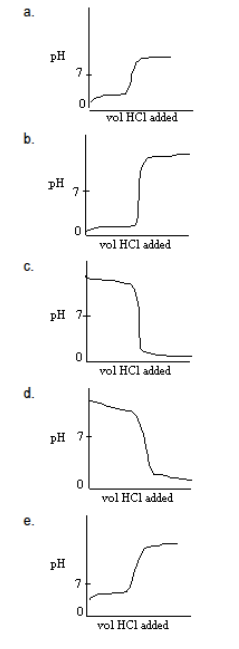# Problem: Which titration curve could describe the titration of a solution of NH2OH by addition of a solution of HNO3?

83% (30 ratings)
###### Problem Details

Which titration curve could describe the titration of a solution of NH2OH by addition of a solution of HNO3?What scientific concept do you need to know in order to solve this problem?

Our tutors have indicated that to solve this problem you will need to apply the Acid and Base Titration Curves concept. You can view video lessons to learn Acid and Base Titration Curves. Or if you need more Acid and Base Titration Curves practice, you can also practice Acid and Base Titration Curves practice problems.

What is the difficulty of this problem?

Our tutors rated the difficulty ofWhich titration curve could describe the titration of a solu...as low difficulty.

How long does this problem take to solve?

Our expert Chemistry tutor, Jules took 2 minutes and 6 seconds to solve this problem. You can follow their steps in the video explanation above.

What professor is this problem relevant for?

Based on our data, we think this problem is relevant for Professor Dungan's class at Trinity Valley Community College.